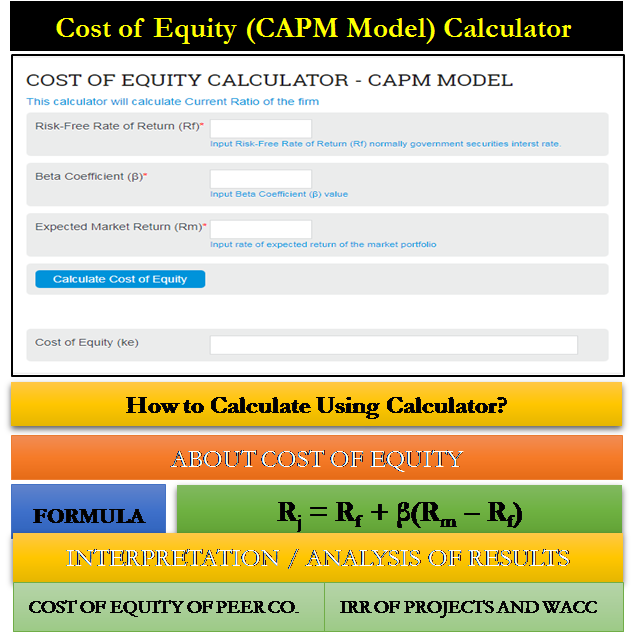data-full-width-responsive="true">

# Cost of Equity (CAPM Model) Calculator

Cost of Equity Calculator (CAPM Model)’ calculates the cost of equity for a company using the formula stated in the Capital Asset Pricing Model.

Cost of equity is the perceptional cost of investing equity capital in a business. Interest is the cost of utilizing borrowed money. For equity, there is no such direct cost available. Therefore, it is calculated based on the general principle of the risk-return trade-off.

## The formula for Cost of Equity using CAPM

The formula for calculating the cost of equity as per CAPM model is as follows:

Rj = R+ β(Rm – Rf)

Rj = Cost of Equity / Required Rate of Return

R= Risk-free Rate of Return. Generally, it is the government’s treasury interest rate. We call it risk-free based on the premises that the government will never default on their financial commitments.

R= Expected Return from Market Portfolio. It is the expected return from an imaginary portfolio of shares where all the shares existing in the market are purchased in the ratio of the market capitalization of each.

β = Beta. It is the measure of risk. It represents the change in return of a particular company in response to a change in the Rm.

## Interpretation / Analysis of Results

First of all, standalone figures of cost of equity of a company have very less relevance. There are no scientific benchmarks for this metric. In general, we can say that lower it is, easier will be the viability of the business. We can get more or better inference when we have following other things along with it.### IRR of Projects and WACC

If we know what is the IRR of the projects of a company, we can calculate WACC of its capital, if it has debt capital too. WACC can be calculated using both costs i.e. equity and debt. Then, we can simply compare the WACC to its IRR. If the IRR is more, the projects are acceptable.

### Cost of Equity of Peer Companies

When the cost of equity of two shares in the same industry is compared, we can find a lot of inferences. If the cost of capital of Company A’s is 18% and Company B is 21%. We can say that the Company B has higher business risk perceived by the market. The management of the company can think on both sides. One, if the risk is really higher in Company B, compared to A. If yes, the company needs to evaluate how they can reduce that risk and lower their cost of capital. On the other hand, there is a possibility that Company B is earning higher profits also against taking higher risks.

Last updated on : October 27th, 2018

** Disclaimer: This post may contain Affiliate Links marked as ** and we may earn a commission on sale.